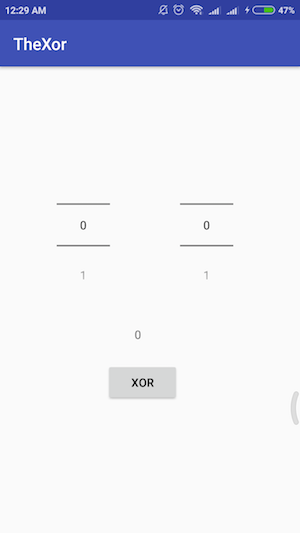# TensorFlow Lite Tutorial -Easy implementation in android

Make apps with the power of deep learning…..

‘You need some working experience on android and some knowledge about basic tensorflow terms(very little)’

Suppose we have a ‘pb’ file of trained TensorFlow * model. I have trained a tensorflow model for simple xor logic gate to keep the deep learning part as simple as possible (As I am weak in maths🙏)

The model takes 2 input of 0 or 1 in each and outputs the probability of output being higher or lower (0 to 1) and from the value we can predict our desired output as 0 or 1 by threshold.

First part of the tutorial we will gather required information from the model (pb ) file and convert it to tensorflow lite model .lite/tflite format. You should have python 3 and tensorflow latest version (Using tensorflow 1.4.1 with python 3.6)environment ready

Conversion Codes : github link

Model file : xorGate.pb

We will load our model in modelinfo.py file and extract our required information from the model file , our output is

Tensor(“import/dense_1_input:0”, shape=(?, 2), dtype=float32)
Tensor(“import/output_node0:0”, shape=(?, 1), dtype=float32)

We have got our required information .

input : ‘dense_1_input’ which will take input in multidimensional array shape of shape=(?, 2) of float32 data type .

output : ‘output_node0’ which will have output in multidimensional array shape of shape=(?, 1) of float32 data type .

Then we will convert the model into tensorflow lite model .

You need bazel installed in your system https://bazel.build/

We need the tensorflow git repository downloaded (link)

From the first tensorflow directory you need to run

``bazel build tensorflow/contrib/lite/toco:toco``

This will compile the toco with bazel (Conversion tool)

Then copy the ‘xorGate.pb’ file in the tensorflow repo root directory`(Which you have downloaded)`

Then run the following command from the tensorflow directory as previous from command line ,input output and shape details are given from the previous output we got

`bazel-bin/tensorflow/contrib/lite/toco/toco \--input_file=xorGate.pb \--input_format=TENSORFLOW_GRAPHDEF \--output_format=TFLITE \--output_file=xorGate.lite \--inference_type=FLOAT \--input_type=FLOAT \--input_arrays=dense_1_input \--output_arrays=output_node0 \--input_shapes=1,2`

As output there will be a xorGate.lite file in the directory which we will use in android.

From here we will start implementing the tflite model in android

Hope you have latest Android Studio installed (using 3.0.1)

Created a new Android project named ‘TheXor’

add tensorflow-lite library using the following line

implementation ‘org.tensorflow:tensorflow-lite:+’

in build.gradle(Module: app) file

add

`android{aaptOptions {    noCompress "tflite"    noCompress "lite"}......`

And sync gradle

create ‘assets’ folder in android app project and copy the xorGate.lite in the folder

Create a simple user interface with two inputs and one output with a buttonUser Interface

Then connect the ui elements with the activity as usual

Use the code below in the activity to load model file

`private MappedByteBuffer loadModelFile(Activity activity,String MODEL_FILE) throws IOException {    AssetFileDescriptor fileDescriptor = activity.getAssets().openFd(MODEL_FILE);    FileInputStream inputStream = new FileInputStream(fileDescriptor.getFileDescriptor());    FileChannel fileChannel = inputStream.getChannel();    long startOffset = fileDescriptor.getStartOffset();    long declaredLength = fileDescriptor.getDeclaredLength();    return fileChannel.map(FileChannel.MapMode.READ_ONLY, startOffset, declaredLength);}`

Import the tensorflow lite interpreter

`import org.tensorflow.lite.Interpreter;`

Then load the model file

`String modelFile="xorGate.lite";Interpreter tflite;`

Load the model file in tensorflow interpreter

`try {    tflite=new Interpreter(loadModelFile(MainActivity.this,modelFile));} catch (IOException e) {    e.printStackTrace();}`

If everything done right up to this point we are very close to test our model

Main part is to get the input and output shape correctly

`float[][] inp=new float[][]{{0,0}};float[][] out=new float[][]{{0}};`

see app source code for better understanding.

Above code is generic we have to get the input value from out two input sources and out should be in right shape according to the information we got from the model description at very beginning.

Its time to do the final step

`tflite.run(inp,out);`

and the results will be saved in out multidimensional array ,the value will be in

`out //array index`

For 0 , 0 input value we will get value in probability not in 0 or 1

As we want the value to be 0 , 1,so we have to round the value and set it to the textview

`result_tv.setText(String.valueOf(Math.round(out)))`

That's it we got our tensorflow model converted in tensorflow-lite and running in Android

Update : With the latest version of tensorflow you can convert model file using python code (link)

App source code : github link

— — — — — — — — — — — — — — — — — — — — — — — — — — — — — — —

Find me:

Github: https://github.com/rdeepc